| Next || Previous || Top |

## TRANSPORT

This keyword data block is used to simulate one-dimensional (1D) transport of solutes, water, colloids, and heat due to the processes of advection and dispersion, diffusion, and diffusion into stagnant zones adjacent to the 1D flow system. Radial and three-dimensional (3D) diffusion can be modeled by using stagnant zones. Multicomponent diffusion allows individual tracer diffusion coefficients to be used in calculating the diffusion of ions; TRANSPORT has capabilities to model multicomponent diffusion in the aqueous solution and in the interlayers of swelling clay minerals. All the chemical processes modeled by PHREEQC, including kinetically controlled reactions, may be included in an advective-dispersive transport simulation. Purely advective transport plus reactions--without diffusion, dispersion, or stagnant zones--can be simulated with the ADVECTION data block.

###### Example data block
`Line 0: TRANSPORT`
`Line 1:	-cells			5`
`Line 2:	-shifts			25`
`Line 3:	-time_step			1 yr 2.0`
`Line 4:	-flow_direction			forward`
`Line 5:	-boundary_conditions			flux constant`
`Line 6:	-lengths			4*1.0 2.0`
`Line 7:	-dispersivities			4*0.1 0.2`
`Line 8:	-correct_disp			true`
`Line 9:	-diffusion_coefficient			1.0e-9`
`Line 10:	-stagnant			1  6.8e-6   0.3   0.1`
`Line 11:	-thermal_diffusion			3.0   0.5e-6`
`Line 12:	-initial_time			1000`
`Line 13:	-print_cells			1-3 5`
`Line 14:	-print_frequency			5`
`Line 15:	-punch_cells			2-5`
`Line 16:	-punch_frequency			5`
`Line 17:	-dump			dump.file`
`Line 18:	-dump_frequency			10`
`Line 19:	-dump_restart			20`
`Line 20:	-warnings			false`
`Line 21:	-multi_D			true	1e-9	0.3	0.05	1.0`
`Line 22:	-interlayer_D		true	0.09	0.01	250 `
```Line 23:	-porosities			4*0.3 0.25
```
```Line 24:	-current			1e-3
```
```Line 25:	-implicit true 			1.0  -30
```
```Line 26:	-same_model 			2-5 8-11
```
###### Explanation

Line 0: TRANSPORT

TRANSPORT is the keyword for the data block. No other data are input on the keyword line.

Line 1: -cells cells

-cells --Indicates the number of cells in the 1D column for the advective-dispersive transport simulation. Optionally, cells or -c [ ells ].

cells --Number of cells in a 1D column. Default is 1.

Line 2: -shifts shifts

-shifts --Indicates the number of shifts or diffusion periods in the advective-dispersive transport simulation. Optionally, shifts or -s [ hifts ].

shifts --For advective-dispersive transport, shifts is the number of advective shifts or time steps, which is the number of times the solution in each cell will be shifted to the next higher or lower numbered cell; the total time simulated is. For purely diffusive transport, shifts is the number of diffusion periods that are simulated; the total diffusion time is. Default is 1.

Line 3: -time_step time_step [unit] [substeps]

-time_step --Defines time step associated with each advective shift or diffusion period. The number of shifts or diffusion periods is given by -shifts . Optionally, timest , -t [ imest ], time_step , or -t [ ime_step ].

time_step --Time (second) associated with each shift or diffusion period. Default is 0 s.

unit --Optional time unit may be second , minute , hour , day , year , or an abbreviation of one of these units. The time_step is converted to seconds after reading the data block; all internal calculations, Basic functions, and output times are in seconds. Default is second.

substeps-- Subdivides the time step into substeps intervals. Used only in multicomponent diffusion calculations, where time_step reduction can help to avoid negative concentrations. The negative concentrations may occur when the time step is too large relative to the explicitly defined stagnant-cell mixing factors; too large a time step causes the Von Neumann criterion to be violated. Default is 1.

Line 4: -flow_direction ( forward , back , or diffusion_only )

-flow_direction --Defines direction of flow. Default is forward at startup. Optionally, direction , flow , flow_direction , -dir [ ection ], or -f [ low_direction ].

forward , back , or diffusion_only --(1) Forward , advective flow direction is into higher numbered cells; optionally, f [ orward ], (2) Backward , advective flow direction is into lower numbered cells; optionally b [ ackward ], or (3) Diffusion_only , only diffusion occurs, there is no advective flow; optionally d [ iffusion_only ] or n [ o_flow ].

Line 5: -boundary_conditions first, last

-boundary_conditions --Defines boundary conditions for the first and last cell. Optionally, bc , bcond , -b [ cond ], boundary_condition , -b [ oundary_condition ]. Three types of boundary conditions are allowed at either end of the column (indicated by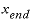):

constant --Concentration is constant, also known as first type or Dirichlet boundary condition. C 0 is the concentration outside the column (mol/kgw). Optionally, co [ nstant ] or 1 .

closed --No flux at boundary, v = 0 and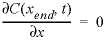, also known as second type or Neumann boundary condition, where v is the flow velocity (m/s, meter per second). Optionally, cl [ osed ] or 2 .

flux --Flux boundary condition,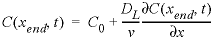, also known as third type or Cauchy boundary condition, where DL is the dispersion coefficient (m2/s). Optionally, f [ lux ] or 3 .

first --Boundary condition at the first cell, constant , closed , or flux . Default is flux .

last --Boundary condition at the last cell, constant , closed , or flux . Default is flux .

Line 6: -lengths list of lengths

-lengths --Defines length of each cell for advective-dispersive transport simulations (m). Optionally, length , lengths , or -l [ engths ].

list of lengths --Length of each cell (m). Any number of lengths up to the total number of cells ( cells ) may be entered. If cells is greater than the number of lengths entered, the final value read will be used for the remaining cells. Multiple lines may be used. Repeat factors can be used to input multiple data with the same value; in the Example data block, 4*1.0 is interpreted as 4 values of 1.0. Default is 1 m.

Line 7: -dispersivities list of dispersivities

-dispersivities --Defines dispersivity of each cell for advective-dispersive transport simulations (m). Optionally, disp , dispersivity , dispersivities , -dis [ persivity ], or -dis [ persivities ].

list of dispersivities --Dispersivity assigned to each cell (m). Any number of dispersivities up to the total number of cells ( cells ) may be entered. If cells is greater than the number of dispersivities entered, the final value read will be used for the remaining cells. Multiple lines may be used. Repeat factors can be used to input multiple data with the same value; in the Example data block, 4*0.1 is interpreted as 4 values of 0.1 m. Default is 0 m.

Line 8: -correct_disp [( True or False )]

-correct_disp --Dispersivity is multiplied by (1 + 1/ cells ) for column ends with flux boundary conditions. This correction can improve modeling effluent composition from column experiments that are modeled with few cells. Default is false at startup. Optionally, correct_disp or -co [ rrect_disp ].

( True or False )-- True indicates that dispersivity is corrected for flux-boundary end cells; false indicates that no correction is made. If neither true nor false is entered on the line, true is assumed. Optionally, t [ rue ] or f [ alse ].

Line 9: -diffusion_coefficient diffusion coefficient

-diffusion_coefficient --Defines diffusion coefficient for all aqueous species (m 2 /s) when not using multicomponent diffusion ( -multi_D false ); this value of the diffusion coefficient is also used as the default thermal diffusion coefficient (see -thermal_diffusion ). Default is 0.3 × 10 -9 m 2 /s at startup. Optionally, diffusion_coefficient , diffc , -dif [ fusion_coefficient ], or -dif [ fc ].

diffusion coefficient --Diffusion coefficient.

Line 10: -stagnant stagnant_cells [ exchange_factor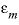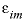]

-stagnant --Defines the maximum number of stagnant (immobile) cells associated with each cell in which advection occurs (mobile cell). Each mobile cell may be connected with up to stagnant_cells immobile cells. The immobile cells associated with a mobile cell are usually conceived to be a 1D column of cells in which solutes from the mobile cell diffuse laterally. However, the connections among the immobile cells can be defined freely with MIX data blocks, which allows calculation of multidimensional diffusion processes (Appelo and Wersin, 2007) and radial diffusion (Appelo and others, 2008, see See Modeling Diffusion of HTO, 36Cl-, 22Na+, and Cs+ in a Radial Diffusion Cell). The immobile cells associated with a mobile cell, cell , are numbered as follows: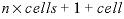, where cells is the number of mobile cells and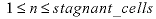. For each immobile cell, a solution (SOLUTION, SOLUTION_SPREAD, or SAVE data block) must be defined, and either a MIX data block or, for the first-order exchange model, the exchange_factor must be defined (only applicable if stagnant_cells equals 1). Mixing will be performed at each diffusion/dispersion time step. EQUILIBRIUM_PHASES, EXCHANGE, GAS_PHASE, KINETICS, REACTION, REACTION_TEMPERATURE, SOLID_SOLUTIONS, and SURFACE may be defined for an immobile cell. Thermal diffusion in excess of hydrodynamic diffusion can be calculated only for the first-order exchange model. Optionally, stagnant or -st [ agnant ].

stagnant_cells --Maximum number of stagnant (immobile) cells associated with a mobile cell. Default is 0.

exchange_factor --Factor describing exchange between a mobile and its immobile cell (s -1 ). The exchange_factor can be used only if stagnant_cells is 1, in which case all immobile cells have the same diffusion properties. WARNING: If exchange_factor is entered, all previously defined MIX structures will be deleted and MIX structures for the first-order exchange model for a dual porosity medium will be created. Default is 0 s -1 .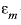--Porosity in each mobile cell, expressed as a fraction of the total volume of mobile and immobile cells. The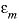is used only if stagnant_cells is 1, in which case all mobile cells have the same porosity. Default is 0 (unitless).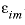--Porosity in each immobile cell, expressed as a fraction of the total volume of mobile and immobile cells. The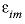is used only if stagnant_cells is 1, in which case all immobile cells have the same porosity. Default is 0 (unitless).

Line 11: -thermal_diffusion temperature retardation factor, thermal diffusion coefficient

-thermal_diffusion --Defines parameters for calculating the diffusive part of heat transport. Diffusive heat transport will be calculated as a separate process if the temperature in any of the solutions of the transport domain differs by more than 1 °C, and when the thermal diffusion coefficient is larger than the effective (aqueous) diffusion coefficient . Otherwise, diffusive heat transport is calculated as a part of aqueous diffusion. The temperature retardation factor , R T , is defined as the ratio of the heat capacity of the total aquifer over the heat capacity of water in the pores,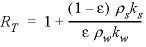, where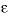is the water filled porosity,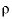is density (kg/m 3 , kilogram per cubic meter), k is specific heat (kJ°C -1 kg -1 ), and subscripts w and s indicate water and solid, respectively. The thermal diffusion coefficient,, can be estimated by using, where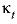is the heat conductivity of the aquifer, including pore water and solid (kJ°C -1 m -1 s -1 , kilojoule per degree Celsius, per meter per second). The value of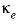may be 100 to 1500 times larger than the aqueous diffusion coefficient, or about 1 × 10 -6 m 2 /s. A temperature change during transport is reduced by the temperature retardation factor (unitless) to account for the heat capacity of the matrix. Optionally, -th [ ermal_diffusion ].

temperature retardation factor --Temperature retardation factor, unitless. Default is 2.0 (unitless).

thermal diffusion coefficient --Thermal diffusion coefficient. Default is the aqueous diffusion coefficient.

Line 12: -initial_time initial_time

-initial_time --Identifier to set the time at the beginning of a transport simulation. The identifier sets the initial value of the variable controlled by -time in the SELECTED_OUTPUT data block. Optionally, initial_time or -i [ nitial_time ].

initial_time --Time (seconds) at the beginning of the transport simulation. Default is the cumulative time including all preceding ADVECTION simulations (for which -time_step has been defined) and all preceding TRANSPORT simulations.

Line 13: -print_cells list of cell numbers

-print_cells --Identifier to select cells for which results will be written to the output file. Optionally, print , print_cells , or -pr [ int_cells ]. Note that the hyphen is required to avoid a conflict with the keyword PRINT.

list of cell numbers --Printing to the output file will occur only for these cell numbers. The list of cell numbers may be continued on the succeeding line(s). A range of cell numbers may be included in the list in the form m-n , where m and n are positive integers, m is less than n , and the two numbers are separated by a hyphen without intervening spaces. Default is 1- cells .

Line 14: -print_frequency print_modulus

-print_frequency --Identifier to select shifts for which results will be written to the output file. Optionally, print_frequency , -print_f [ requency ], output_frequency , or -o [ utput_frequency ].

print_modulus --Printing to the output file will occur for advection shifts or diffusion periods that are evenly divisible by print_modulus . Default is 1.

Line 15: -punch_cells list of cell numbers

-punch_cells --Identifier to select cells for which results will be written to the selected-output file. Optionally, punch , punch_cells , -pu [ nch_cells ], selected_cells , or -selected_c [ ells ].

list of cell numbers --Printing to the selected-output file will occur only for these cell numbers. The list of cell numbers may be continued on the succeeding line(s). A range of cell numbers may be included in the list in the form m-n , where m and n are positive integers, m is less than n , and the two numbers are separated by a hyphen without intervening spaces. Default is 1- cells .

Line 16: -punch_frequency punch_modulus

-punch_frequency --Identifier to select shifts for which results will be written to the selected-output file. Optionally, punch_frequency , -punch_f [ requency ], selected_output_frequency , -selected_o [ utput_frequency ].

punch_modulus --Printing to the selected-output file will occur for advection shifts or diffusion periods that are evenly divisible by punch_modulus . Default is 1.

Line 17: -dump dump file

-dump --Identifier to write a complete state of the advective-dispersive transport simulation to dump file after every dump_modulus advection shifts or diffusion periods. The file is formatted as an input file that can be used to restart calculations. Previous contents of the file are overwritten each time the file is written. Optionally, dump or -du [ mp ].

dump file --Name of the file to which complete state of the advective-dispersive transport simulation will be written. Default is phreeqc.dmp .

Line 18: -dump_frequency dump_modulus

-dump_frequency --Complete state of the advective-dispersive transport simulation will be written to the dump file for advection shifts or diffusion periods that are evenly divisible by dump_modulus . Optionally, dump_frequency or -dump_f [ requency ].

dump_modulus -- Number of advection shifts or diffusion periods. Default is shifts /2 or 1, whichever is larger.

Line 19: -dump_restart shift number

-dump_restart --If an advective-dispersive transport simulation is restarted from a dump file, the starting shift number is given on this line. Optionally, dump_restart or -dump_r [ estart ].

shift number --Starting shift number for the calculations, if restarting from a dump file. The shift number is written in the dump file by PHREEQC. It equals the shift number at which the dump file was created. Default is 1.

Line 20: -warnings [( True or False )]

-warnings --Identifier enables or disables printing of warning messages for transport calculations. In some cases, transport calculations could produce many warnings, which are not errors. Once it is determined that the warnings are not due to erroneous input, disabling the warning messages can avoid generating large output files. Default is true at startup. Optionally, warnings , warning , or -w [ arnings ].

( True or False )--If true , warning messages are printed to the screen and the output file; if false , warning messages are not printed to the screen nor the output file. The value set with -warnings is retained in all subsequent transport simulations until changed. If neither true nor false is entered on the line, true is assumed. Optionally, t [ rue ] or f [ alse ].

Line 21: -multi_D ( True or False ) default_Dw porosity porosity_limit Archie_n

-multi_D --Enables or disables the calculation of multicomponent diffusion. In multicomponent diffusion each solute can be given its own diffusion coefficient, allowing it to diffuse at its own rate, but with the constraint that overall charge balance is maintained (Vinograd and McBain, 1941; Appelo and Wersin, 2007), Optionally, multi_D or -m [ ulti_D ] (as with all identifiers, case insensitive). With -multi_D true , the diffusive flux is calculated by (see also Notes):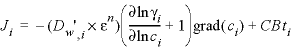,

where i indicates the species; Ji is the flux (mol m -2 s -1 , mole per square meter per second);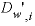is the (temperature corrected) tracer diffusion coefficient (m 2 /s); ε is the water-filled (or accessible) porosity (unitless); n is an empirical exponent, known from Archie’s law to be about 1; γ i is the activity-coefficient (unitless); ci is the concentration (mol/m 3 , mole per cubic meter); grad( ci ) is the concentration gradient (mol/m 4 , mole per meter to the fourth power) , which may be different in free (uncharged) pore water and in the Donnan pore space on a surface (see Notes); and CBti is the charge balance term (Appelo and Wersin, 2007, see the Notes). The tracer diffusion coefficients are defined with keyword SOLUTION_SPECIES in phreeqc.dat for 25 °C, and corrected to temperature T (K) of the solution as follows:

D w ' = (D w )298 ×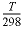×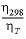,

where η is the viscosity of water.

When -multi_D is false , the diffusive flux is calculated with J i = - D p × grad( c i ), where D p is the same for all species (defined with identifier -diffusion_coefficient as in Line 9) and not corrected for changes of temperature.

Note that PHREEQC assumes that, for diffusion, the cell contains water exclusively, and uses the pore water diffusion coefficient for calculating the flux. (The effective diffusion coefficient (D e ) is for a volume of grains and pores together, and is related to D p as follows: D e = D p ε ). The identifier -stagnant allows for nonuniform porosities, nonuniform tortuosities, and other variations in the diffusion domain, provided mixing factors among cells are defined explicitly in the input file with MIX data blocks.

( True or False )--If true , multicomponent diffusion is calculated; if false , diffusion is calculated with the diffusion coefficient given in Line 9 .

default_Dw --The diffusion coefficient (m 2 /s at 25 °C) given to solute species for which -dw is not defined in keyword SOLUTION_SPECIES . T he value must be used when calculating explicit mixing factors for stagnant cells. Default is 0 m 2 /s.

porosity --The porosity filled with free and Donnan pore water in the cells; the porosity is a fraction of a representative volume of the porous medium (unitless). Initially all cells are defined with the same porosity . The porosity for a cell can be changed in any keyword data block that supports Basic programming (RATES, USER_GRAPH, USER_PRINT, and USER_PUNCH) by using the PHREEQC Basic function CHANGE_POR(porosity, cell_no). The porosity in a cell can be retrieved with the function GET_POR(cell_no). Default is 0 (unitless).

porosity_limit --The porosity limit, below which diffusion stops. Default is 0 (unitless).

Archie_n --The exponent n used for calculating the pore-water diffusion coefficient (D p, i ) from the tracer diffusion coefficient (D w, i ), Dp, i = Dw, i ε n, where ε is the water filled (or the accessible) porosity (unitless), and n is an empirical exponent that varies from approximately 0.9 to 1.2 (Grathwohl, 1998; Van Loon and others, 2007) but may be higher for diffusion perpendicular to the bedding plane. The parameter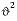(approximately 1 / ε wn) is the tortuosity factor, which accounts for the longer diffusion path for a particle in a porous media than in pure water.

Line 22: -interlayer_D ( True or False ) interlayer_porosity interlayer_porosity_limit interlayer_tortuosity_factor

-interlayer_D --Enables or disables the calculation of interlayer diffusion in swelling clay minerals. If -interlayer_D is true , -multi_D also must be true , and the -multi_D parameters must be set as explained with Line 21. Optionally, interlayer_D or -int [ erlayer_D ] (as with all identifiers, case insensitive). The flux in the interlayers is calculated for the cations associated with X- (as defined with keyword EXCHANGE):
Ji = -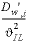× mCEC × grad( βι ) + CBti,

where, i indicates an aqueous species, Ji is the flux (mol m -2 s -1 ), Dw',i is the temperature corrected diffusion coefficient,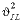is the interlayer tortuosity factor (unitless), mCEC is the concentration of total X-, mol(X-) / (m3 interlayer water), where (m3 interlayer water) = (m3 free pore water + m3 Donnan water) × ( εIL / ε ), grad( βι ) is the gradient of the equivalent fraction of species i on the exchange sites (1/m), and CBti is the charge balance term (Appelo and Wersin, 2007). The tracer diffusion coefficients are defined with keyword SOLUTION_SPECIES in phreeqc.dat for 25 °C and are corrected to temperature T (K) of the solution with the equation Dw' = (Dw )298 ××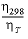, where η is the viscosity of water.

( True or False )--If true , interlayer diffusion is calculated; if false , interlayer diffusion is not calculated .

interlayer_porosity --The porosity of interlayer water, a fraction of the total volume . Default is 0 (unitless) .

interlayer_porosity_limit --The porosity of interlayer water, below which interlayer diffusion stops. Default is 0 (unitless).

interlayer_tortuosity_factor --The tortuosity factor for interlayer diffusion,(unitless). Default is 100.0.

Line 23: -porosities list of porosities

-porosities--Defines porosity of each cell for diffusive transport simulations (m). Optionally, po[rosities] or -po[rosity].

list of porosities--Porosity of each cell (unitless). Any number of porosities up to the total number of cells (cells) may be entered. If cells is greater than the number of lengths entered, the final value read will be used for the remaining cells. Multiple lines may be used. Repeat factors can be used to input multiple data with the same value; in the Example data block, 4*0.30 is interpreted as 4 values of 0.30. The values entered here take pecedence ofver the value given with -multi_D. If one stangant layer is defined together with an exchange-factor > 0 (‘-stagnant 1 4e-6 0.3 0.1’), the mobile (here = 0.3) and immobile (here = 0.1) porosities defined with -stagnant are used. If -interlayer_d is defined, then the total porosity for a cell is the sum of the porosity defined here and the porosity defined by -interlayer_d.

Line 24: -fix_current current

-fix_current--Current in a column experiment. Default is 0 at startup. Optionally, fix_current, -fi[x_current], or current, or -cu[rrent].

current--Electrical current, Default is 0 amperes.

Line 25: -implicit (True or False) max_mix_factor [min_LM]

-implicit--Select implicit algorithm that allows for for large time steps when multicomponent diffusion is calculated. Optionally, implicit or -im[plicit].

(True or False)--True, implicit method or false, explicit method is used for multicomponent diffusion calculations.

max_mix_factor--Maximum mix factor allowed, where max_mix_factor = D * Δt / Δx2. The default value of max_mix_factor = 1, but it can be increased often to > 4, depending on the required accuracy and the chemistry of the system, and the calculations will be about an order of magnitude faster.With implicit true, electro-migration calculation is more stable and usually avoids the (possibly) disturbing warnings of ‘negative moles’. The implicit calculations have been implemented for a regular column and, optionally a single stagnant cell. The implicit method is not implemented for interlayer diffusion.

min_LM--Defines a minimum log10 molality for including a species in multicomponent diffusion calculations. If log10 concentration of an aqueous species is less than this value in all cells, then the species is not included in the multicomponent diffusion calculation. Default is -30; a value of -12 is reasonable.

Line 26: -same_model cell ranges

-same_model--Use the chemical model structure of the previous calculation for specific cells Optionally, same_model or -sa[me_model].

cell ranges--One or more ranges of cell numbers, where a range is either a single cell number or the beginning and ending cell number separated by a hyphen. The composition of the cells in a range are set to the values of the preceding cell number. For example, -same_model 2-5 8-11 use for cells 2-5 the model defined for cell 1, and for cells 8-11 the model defined for cell 7.

###### Notes

The advective-dispersive transport capabilities of PHREEQC are derived from a formulation of 1D, advective-dispersive transport presented by Appelo and Postma (2005). The 1D column is defined by a series of cells (number of cells is cells ), each of which has the same pore volume. Lengths are defined for each cell and the time step ( time step ) gives the time necessary for a pore volume of water to move through each cell. Thus, the velocity of water in each cell is determined by the length of the cell divided by the time step. In the Example data block, a column of five cells ( cells ) is modeled and 5 pore volumes of filling solution are moved through the column ( shifts / cells is 5). The total time of the simulation is 25 yr (year) (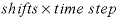). The total length of the column is 6 m (four 1-m cells and one 2-m cell).

At each shift, advection is simulated by moving solution cells - 1 to cell cells , solution cells - 2 to cell cells - 1, and so on, until solution 0 is moved to cell 1 (upwind scheme). With flux-type boundary conditions, the dispersion steps follow the advective shift. With Dirichlet boundary conditions, the dispersion step and the advective shift are alternated. After each advective shift and dispersion step, kinetic reactions and chemical equilibria are calculated. The moles of pure phases and the compositions of the exchange assemblage, surface assemblage, gas phase, solid-solution assemblage, and kinetic reactants in each cell are updated after each chemical calculation.

For advective transport, the influent solution must be defined, otherwise the program stops with an error message. Solution 0 is the influent with -flow_direction forward ; solution cells + 1 is the influent when the flow is backward . If the effluent (solution cells + 1, for direction forward ) is not defined, the program copies it from the effluent boundary cell in the column. A closed boundary condition is not possible with advective flow, and the boundary condition will be changed to a flux boundary condition. Likewise, a flux-boundary condition is not possible when pure diffusion is modeled, and the boundary condition will be changed to a closed boundary condition.

The -time_step identifier defines the length of time associated with each advective shift or diffusion period. The program may subdivide this time step into smaller dispersion time steps if necessary to calculate dispersion accurately. Each dispersion time step may be further subdivided to integrate the kinetic reactions (KINETICS data block). Kinetic reactions are likely to slow the calculations by a factor of six or more compared to pure equilibrium calculations.

The numerical scheme is for cell-centered concentrations, which has consequences for data interpretation. Thus, the composition in a boundary cell is a half-cell distance away from the column outlet and needs a half time step to arrive at (or from) the column end. The half time step must be added to the total residence time in the column when effluent from a column is simulated [use (TOTAL_TIME + time step / 2) for time, see See 1D Transport: Kinetic Biodegradation, Cell Growth, and Sorption, or ((STEP_NO + 0.5) / cells ) for pore volumes, see See Transport and Cation Exchange]. The kinetics time for advective transport into the boundary cell is the advective time step divided by 2. Also, the cell-centered scheme does not account for dispersion in the border half-cell in case of a flux boundary condition. The identifier -correct_disp provides an option to correct the ignored dispersion by increasing the dispersivity for all cells in the column by the appropriate amount. The correction will improve the comparison with analytical solutions for conservative elements when the number of cells is small.

A “dual porosity” model, in which part of the porosity allows advective flow and part of the porosity is accessible only by diffusion, can be developed with a first-order exchange model or with finite differences, and both approaches can be defined in terms of a mixing among cells (see “Transport in Dual Porosity Media” in Parkhurst and Appelo, 1999). With the TRANSPORT data block, one column of mobile cells is used to represent the part of the flow system in which advection occurs, and then additional immobile cells connected to the mobile cells are used to represent the stagnant zone that is accessible only by diffusion. The stagnant zone can be defined to be parallel or perpendicular to the column of mobile cells or to be a combination of the two by proper definition of mixing factors in MIX data blocks. A shortcut is available for the classical formulation of a dual porosity medium with a first-order rate of exchange. In this case, -stagnant is used to define one stagnant cell for each mobile cell ( stagnant_cells = 1), an exchange factor ( exchange_factor ) for the exchange between immobile and mobile cells, and the porosities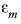and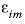for the mobile and immobile cells.

Thermal diffusion can be modeled for a stagnant zone with first-order exchange between mobile and immobile cells. Thermal exchange is calculated after subtracting the part of the exchange that is associated with hydrodynamic diffusion (see “Transport of Heat” in Parkhurst and Appelo, 1999). PHREEQC uses the value of the diffusion coefficient to find the correct heat exchange factor, and the value entered with identifier -diffusion_coefficient should be the same as has been used to calculate the exchange factor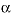(see equation 125 in Parkhurst and Appelo, 1999).

Most of the information for advective-dispersive transport calculations must be entered with other keyword data blocks. Advective-dispersive transport assumes that solutions with numbers 1 through cells have been defined by using SOLUTION, SOLUTION_SPREAD, or SAVE data blocks. In addition the infilling solution must be defined. If -flow_direction is forward , solution 0 is the infilling solution; if -flow_direction is backward , solution cells + 1 is the infilling solution, if -flow_direction is diffusion_only , then infilling solutions at both column ends are optional. If stagnant zones are modeled, solution compositions for the stagnant-zone cells must be defined with SOLUTION, SOLUTION_SPREAD, or SAVE data blocks.

Pure-phase assemblages may be defined with EQUILIBRIUM_PHASES or SAVE, with the number of the assemblage corresponding to the cell number. Likewise, an exchange assemblage, a surface assemblage, a gas phase, or a solid-solution assemblage can be defined for each cell through EXCHANGE, SURFACE, GAS_PHASE, SOLID_SOLUTIONS, or SAVE keywords, with the identifying number corresponding to the cell number. Kinetically controlled reactions can be defined for each cell through the KINETICS data block. Note that ranges of numbers can be used to define multiple solutions, exchange assemblages, surface assemblages, gas phases, solid-solution assemblages, or kinetic reactions simultaneously and that SAVE allows definition of a range of numbers. Constant-rate reactions can be defined for mobile or immobile cells through REACTION data blocks, again with the identifying number of the REACTION data block corresponding to the cell number. REACTION_TEMPERATURE data blocks can be used to specify the initial temperatures of the cells in the transport simulation. Temperatures in the cells may change during the transport simulation depending on the temperature distribution and the temperature retardation factor defined by -thermal_diffusion . REACTION_PRESSURE data blocks can be used to set the pressure in each cell.

By default, the composition of the solution, pure-phase assemblage, exchange assemblage, surface assemblage, gas phase, solid-solution assemblage, and kinetic reactants are printed for each cell for each shift. Use of -print_cells and -print_frequency will limit the amount of data written to the output file. If -print_cells has been defined then only the specified cells will be written; otherwise, all cells will be written. The identifier -print_frequency will restrict writing to the output file to those shifts that are evenly divisible by print_modulus . In the Example data block, results for cells 1, 2, 3, and 5 are written to the output file after each integer pore volume (5 shifts) has passed through the column. Data written to the output file can be further limited with the keyword PRINT (see -reset false ).

If a SELECTED_OUTPUT data block has been defined, then selected data are written to the selected-output file. Use of -punch_cells and -punch_frequency in the TRANSPORT data block will limit the data that are written to the selected-output file. If -punch_cells has been defined then only the specified cells will be written; otherwise, all cells will be written. The identifier -punch_frequency will restrict writing to the selected-output file to those shifts that are evenly divisible by punch_modulus . In the Example data block, results are written to the selected-output file for cells 2, 3, 4, and 5 after each integer pore volume (5 shifts) has passed through the column.

At the end of an advective-dispersive transport simulation, all the physical and chemical data (for example, compositions of solutions, equilibrium-phase assemblages, surfaces, exchangers, solid solutions, and kinetic reactants) are automatically saved and are identified by the cell number in which they reside. These data are available for subsequent simulations within a single run. Transient conditions can be simulated by including subsequent SOLUTION and TRANSPORT data blocks, which may define new chemical boundary and transport conditions. Only parameters that differ from the previous advective-dispersive transport simulation need to be redefined, such as new infilling solution (SOLUTION 0), a change from advection to diffusion only ( -flow_direction diffusion_only ), or a change in flow direction from forward to backward ( -flow_direction backward ). All parameters not specified in the new TRANSPORT data block remain the same as the previous advective-dispersive transport simulation. Normally, the diffusion coefficient, lengths of cells, dispersivities, and stagnant zone definitions remain the same through all advective-dispersive transport simulations and thus need not be redefined.

For long advective-dispersive transport calculations, it may be desirable to save intermediate states in the calculation, either because of hardware failure or because of nonconvergence of the numerical method. The -dump_frequency identifier allows intermediate states to be saved at intervals during the calculation. The -dump identifier allows the definition of a file name in which to write these intermediate states. The dump file is formatted as an input file for PHREEQC, so calculations can be resumed from the point at which the dump file was made. The -dump_restart identifier allows a shift number to be specified from which to restart the calculations.

With the identifiers -multi_D and -interlayer_D , diffusion can be calculated as a multicomponent process in uncharged (“free”) pore water, in electrostatic double layer (EDL, referred to as the Donnan pore space) water at charged surfaces, and in interlayer water of swelling clay minerals. Each solute species or surface defined with -dw greater than zero diffuses at its own speed, while overall, charge balance is maintained (Vinograd and McBain, 1941; Appelo and Wersin, 2007). Instead of concentration, as in Fick’s laws, the thermodynamic potential forms the basis for calculating the multicomponent flux:

### μi = μi0 + RT ln ai + ziFψ, (9)

where μi0 is the standard thermodynamic potential of species i (J/mol, joule per mole), R is the gas constant (8.314 J K-1mol-1), T is the absolute temperature (K), ai is the activity (unitless), zi is charge number (unitless), F is the Faraday constant (96485 J V-1eq-1), and ψ is the electrical potential (V). The activity is related to concentration ci (here, mol/m3) by ai = γi ci/c0, where γi is the activity coefficient (unitless) and c0 is the standard state (here 1.0 mol/m3). The diffusive flux of i as a result of chemical and electrical potential gradients is

###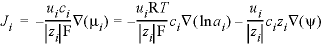, (10)

where Ji is the flux of species i (mol m-2s-1), ui is the mobility (m2 s-1V-1, square meter per second per volt), ci is the concentration (mol/m3), zi is charge number (unitless),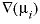is the gradient of the chemical potential (J mol-1m-1, joule per mole per meter), and similarly for the gradients of lnai and ψ. If the electrical current is zero,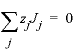, equation 10 can be rearranged to solve for the gradient of the electrical potential:

###, (11)

where the variables are linked with species j to indicate their origin from the zero-charge transfer condition. With ai = γi ci/c0 and cid(lnci) = d(ci), the gradient of the activity becomes:

###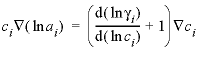. (12)

And, finally, using the identity

###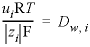, (13)

equation 10 can be recast completely in known model variables:

###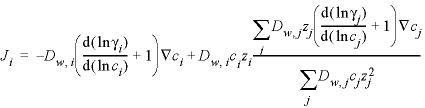. (14)

Appelo and Wersin (2007) have shown that the thermodynamic potential gradients are the same in free pore water and in EDL water (the Donnan pore space); only the concentrations are different in the two water types. The concentration in the Donnan pore space is:

###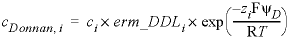, (15)

where erm_DDLi is the enrichment factor in the Donnan pore space, defined with identifier -erm_ddl in keyword SOLUTION_SPECIES, and ψD is the potential in the Donnan pore space. Accordingly, for calculating the flux through the Donnan space, if -only_counter_ions is false , ci in equation 14 is replaced by cDonnan, i for the fraction of Donnan water in the pore space. If -only_counter_ions is true , the concentration gradient in free pore water is used for counter-ions (ci in equation 14 is not replaced for counter-ions), and for co-ions the flux is zero (their concentration is zero), see below.

Diffusion of exchangeable cations can be calculated when they are defined with exchange species X - , as in the databases phreeqc.dat and wateq4f.dat. The concentration of an exchangeable species is

### ci = γι × βι × CEC / zi, (16)

where ci is the concentration of species i (mol/m3 interlayer water), γι is the activity coefficient, defined with -gamma in keyword EXCHANGE_SPECIES, β is the equivalent fraction of the exchangeable species, and CEC is the exchange capacity (eq/m3, equivalent per cubic meter of interlayer water), defined as moles X- in keyword EXCHANGE (the volume of interlayer water is calculated from the interlayer porosity, as explained below). For calculating the flux through the interlayer space,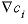in equation 14 is replaced by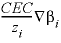.

The actual mass transfer is found by multiplying the flux by the surface area, which PHREEQC calculates either from the amount of water in a cell and the cell length in a regular column, or from the mixing factor defined for stagnant cells, assuming a density of water of 1 kg/L. Thus, in a regular column, the surface area for diffusion between two cells i and j is

###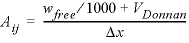, (17)

where Aij is the surface area (m2), wfree is kgw defined with -water in keyword SOLUTION, VDonnan is the volume of water in the Donnan pore space, equal to Asurf × tDonnan, the product of the area of the surface (m2) and the thickness of the Donnan layer (m), both defined in keyword SURFACE, and Δx is the cell length (m) defined with -lengths .

For stagnant cells, the mixing factor is multiplied by the ratio of the diffusion coefficient of a species and the default diffusion coefficient that is used when calculating the mixing factor. The mixing factor is given by equation 128 of the PHREEQC version 2 manual (Parkhurst and Appelo, 1999) or equation S2 of Appelo and Wersin, 2007):

###, (18)

where mixf ij is the mixing factor between cells i and j, defined with keyword MIX. D p is the pore water diffusion coefficient, given by D p = D w ε n , where D w is the default diffusion coefficient; ε is the porosity; and n is Archie’s factor, all defined with identifier -multi_D . Δt is the time step defined with -time_step . f bc is a correction factor that equals 2 for constant concentration boundary cells and is 1 otherwise, hij is the distance between the midpoints of cells i and j, and Vj is the volume of pore water in cell j for which the mass transfer is calculated. The mixfij that is defined in the input file must be calculated with a volume Vj of 0.001 m3. PHREEQC will adapt the value of mixfij by using the actual amount of water in the cell, which is given by (w free /1000 + VDonnan). Furthermore, (according to equation 18) the mixing factor for individual species is multiplied by D w, i /D w .

For interlayer diffusion, PHREEQC calculates the surface area by

###, (19)

where εIL is the porosity occupied by interlayer water, defined with identifier -interlayer_D , and ε is the porosity of free and Donnan pore water together, defined with -multi_D . For stagnant cells, the mixing factor is multiplied with the ratio of the interlayer porosity and the free and Donnan porosity, and with the ratio of the diffusion coefficients, and of the inverse tortuosity coefficients. If the surface areas and (or) the porosities are different for the two cells, the harmonic mean is used (see See Modeling Diffusion of HTO, 36Cl-, 22Na+, and Cs+ in a Radial Diffusion Cell, in Examples).

For the Donnan pore space, the enhanced concentration gradient for counter-ions and the decreased concentration gradient for co-ions is applied if identifier -only_counter_ions is false in keyword SURFACE. If identifier -only_counter_ions is true , the concentration of the co-ions is zero in the Donnan space, and also the flux of the co-ions is zero. In this case, the concentration gradient of counter-ions in free pore water is used for the Donnan pore space for two reasons. First, because with only counter ions in the Donnan pore space, the concentrations of the counter-ions will be smaller than in free pore water if the surface charge is smaller than the charge of the co-ions in solution. This could give unrealistic gradients when surface charges are different from cell to cell. The second reason is that it allows direct comparison of multicomponent results of PHREEQC with traditional calculations in which Fick’s laws are used to model the behavior of individual tracers in clays and clay-containing rocks.

###### Example problems

The keyword TRANSPORT is used in example problems 11, 12, 13, 15, and 21. Examples of multicomponent diffusion are given in Appelo and Wersin (2007), supplementary information, Appelo and others (2008) and (2010), and in http://www.hydrochemistry.eu/exmpls/index.html#new (accessed June 25, 2012). An example of interlayer diffusion is available in http://www.hydrochemistry.eu/exmpls/opa_col.html#new2 (accessed June 25, 2012).

###### Related keywords

| Next || Previous || Top |